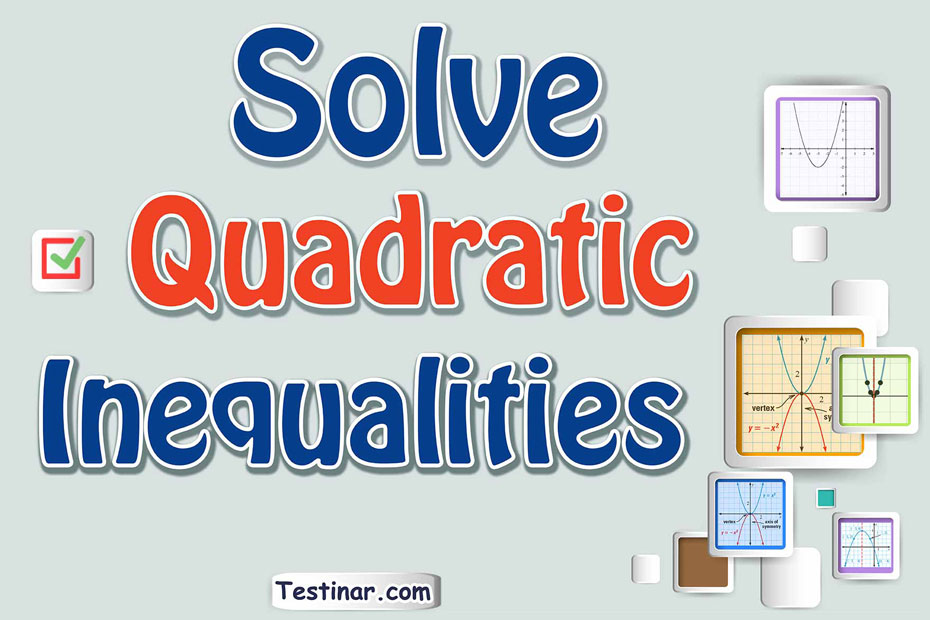## How to Solve Quadratic Inequalities

A quadratic inequality has two roots, or x-intercepts because it has an $$x^2$$ term. You get a parabola when you plot this inequality on a coordinate plane. Finding the values of $$x$$ that make the inequality true is what it means to solve an inequality. By drawing the inequality, you can show these solutions in algebra or on a number line or coordinate plane.

### Follow these steps to figure out how to solve a quadratic inequality:

• Step1:
Solve the inequality as if it were an equation.
• Step2:
The boundary points for the solution to the inequality are the real answers to the equation.
• Step3:
If the original inequality did not include equality, make the boundary points open circles. If it did, make the boundary points solid circles.
• Step4:
Choose points from each of the areas that the boundary points make. Change these "test points" in the original inequality.
• Step5:
If a test point meets the original inequality, then the area that contains that test point is part of the solution.
• Step6:
Show the solution both as a picture and as a solution set.

### Example:

Solve $$(x \ + \ 1) \ (2x \ - \ 4) \ < \ 0$$.

Figure out $$(x \ + \ 1) \ (2x \ - \ 4) \ = \ 0$$. Using the zero-product property,

$$(x \ + \ 1) \ = \ 0$$ or $$(2x \ - \ 4) \ = \ 0$$ $$⇒ \ x \ = \ -1 \ , \ x \ = \ 2$$

Make the points of the border. Here, the boundary points are open circles because the original inequality doesn't include equality.

Points of the border: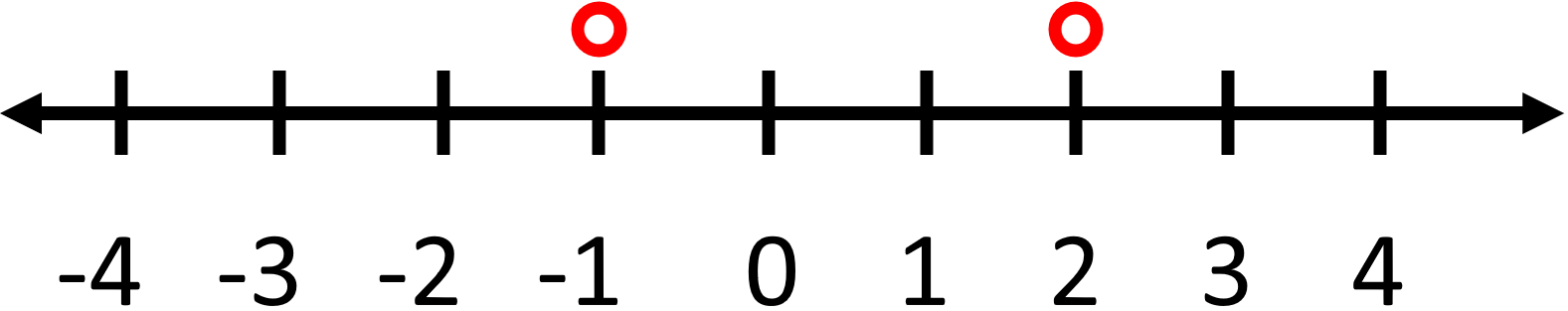There are now three different areas: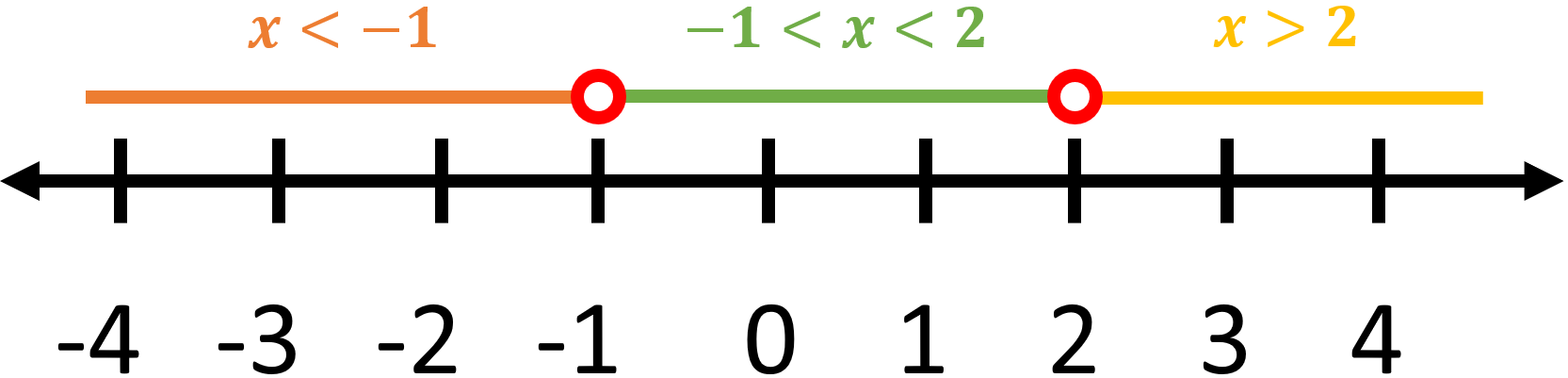Choose test points from these three areas: $$x \ = \ -3 \ , \ x \ = \ 0 \ , \ x \ = \ 3$$

Check to see if the test points meet the first inequality.

• $$x \ = \ -3 \ ⇒ \ (-3 \ + \ 1) \ (2(-3) \ - \ 4) \ < \ 0 \ ⇒ \ (-2)(-10) \ < \ 0 \ ⇒ \ 20 \ < \ 0$$
• $$x \ = \ 0 \ ⇒ \ (0 \ + \ 1) \ (2(0) \ - \ 4) \ < \ 0 \ ⇒ \ (1)(-4) \ < \ 0 \ ⇒ \ -4 \ < \ 0$$
• $$x \ = \ 3 \ ⇒ \ (3 \ + \ 1) \ (2(3) \ - \ 4) \ < \ 0 \ ⇒ \ (4)(2) \ < \ 0 \ ⇒ \ 8 \ < \ 0$$

Since $$x \ = \ 0$$ solves the original problem, the area $$-1 \ < \ x \ < \ 2$$ is part of the answer. Since $$x \ = \ -3$$ doesn't solve the original problem, the area $$x \ < \ -1$$ isn't part of the answer. Since $$x \ = \ 3$$ doesn't solves the original problem, the area $$x \ > \ 2$$ isn't part of the answer. Now show the solution in a graphic form and a solution set.

The graphic form: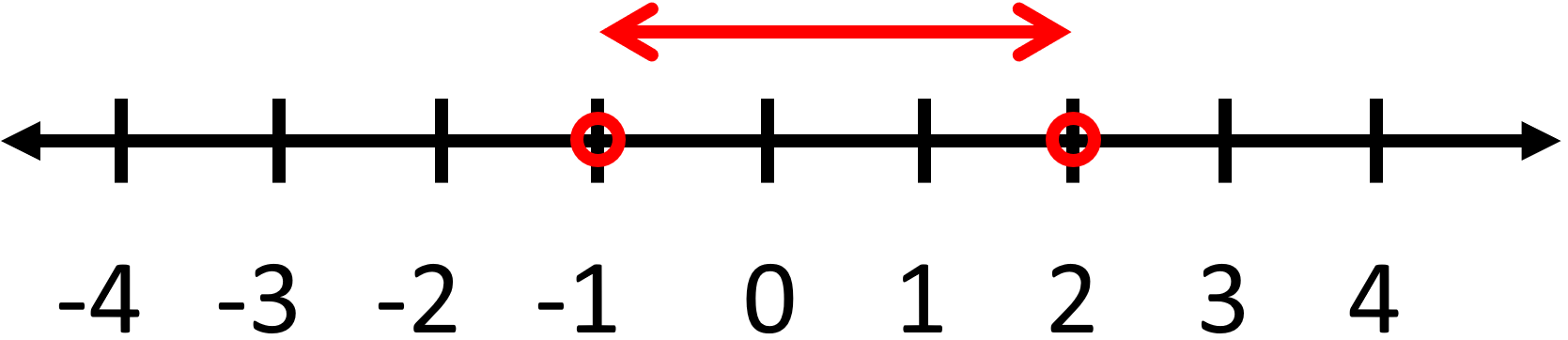The form of the solution set: $$\{x \ | \ -1 \ < \ x \ < \ 2\}$$

### Exercises for Solving Quadratic Inequalities

1) Solve: $$(2x \ + \ 2)(3x \ - \ 6) \ > \ 0$$

2) Solve: $$(2x \ + \ 6)(3x \ - \ 12) \ < \ 0$$

3) Solve: $$(-2x \ + \ 6)(4x \ + \ 4) \ < \ 0$$

4) Solve: $$2x^2 \ - \ 10x \ + \ 8 \ < \ 0$$

5) Solve: $$3x^2 \ + \ 12x \ + \ 9 \ > \ 0$$

6) Solve: $$x^2 \ - \ 1 \ > \ 0$$

7) Solve: $$x^2 \ - \ 4 \ < \ 0$$

8) Solve: $$(2x \ + \ 2)(3x \ - \ 6) \ < \ 0$$

9) Solve: $$x^2 \ - \ x \ - \ 12 \ > \ 0$$

10) Solve: $$(-x \ - \ 1)(x \ + \ 3) \ > \ 0$$

1) Solve: $$(2x \ + \ 2)(3x \ - \ 6) \ > \ 0$$

$$\color{red}{x_1 \ = \ -1, \ x_2 \ = \ 2}$$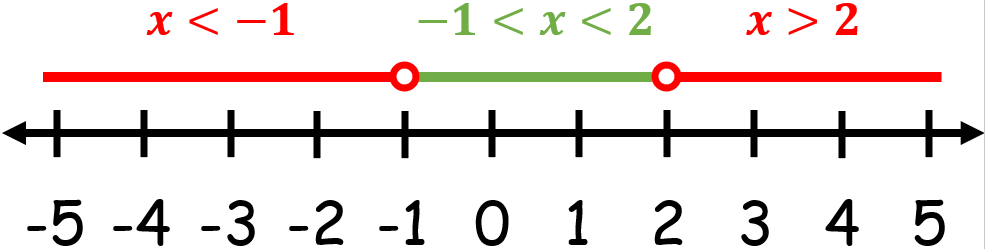Test points: $$\color{red}{x \ = \ -2, \ x \ = \ 0, x \ = \ 3}$$
So, $$\color{red}{⇒ \ x \ < \ -1, \ x \ > \ 2}$$ is the solution.
Solution set: $$\color{red}{\{x \ | \ x \ < \ -1, \ x \ > \ 2\}}$$

2) Solve: $$(2x \ + \ 6)(3x \ - \ 12) \ < \ 0$$

$$\color{red}{x_1 \ = \ -3, \ x_2 \ = \ 4}$$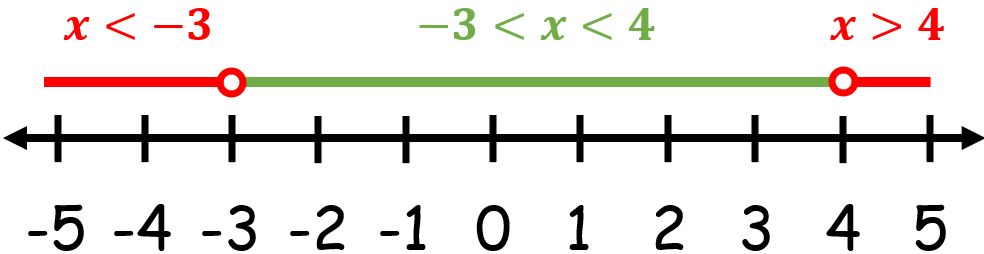Test points: $$\color{red}{x \ = \ -4, \ x \ = \ 0, x \ = \ 5}$$
So, $$\color{red}{⇒ \ -3 \ < \ x \ < \ 4}$$ is the solution.
Solution set: $$\color{red}{\{x \ | \ -3 \ < \ x \ < \ 4\}}$$

3) Solve: $$(-2x \ + \ 6)(4x \ + \ 4) \ < \ 0$$

$$\color{red}{x_1 \ = \ 3, \ x_2 \ = \ -1}$$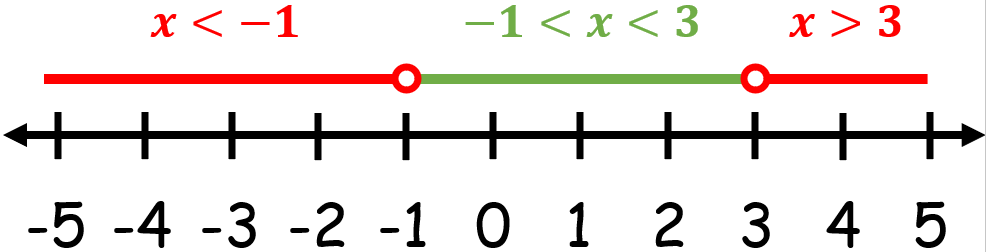Test points: $$\color{red}{x \ = \ -2, \ x \ = \ 0, x \ = \ 4}$$
So, $$\color{red}{⇒ \ x \ < \ -1, \ x \ > \ 3}$$ is the solution.
Solution set: $$\color{red}{\{x \ | \ x \ < \ -1, \ x \ > \ 3\}}$$

4) Solve: $$2x^2 \ - \ 10x \ + \ 8 \ < \ 0$$

$$\color{red}{x_1 \ = \ 1, \ x_2 \ = \ 4}$$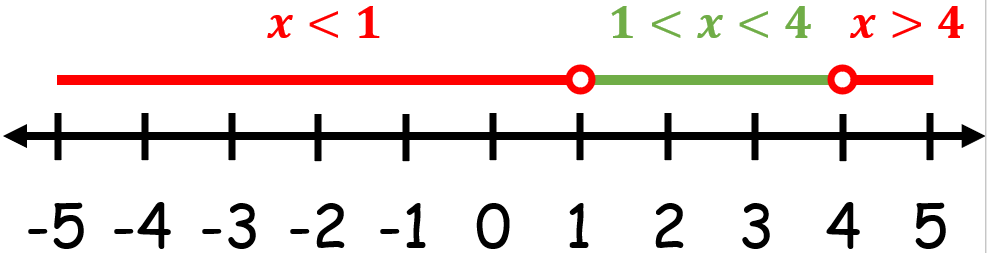Test points:
$$\color{red}{x \ = \ 0, \ x \ = \ 2, x \ = \ 5}$$
So, $$\color{red}{⇒ \ 1 \ < \ x \ < \ 4}$$ is the solution.
Solution set: $$\color{red}{\{x \ | \ 1 \ < \ x \ < \ 4\}}$$

5) Solve: $$3x^2 \ + \ 12x \ + \ 9 \ > \ 0$$

$$\color{red}{x_1 \ = \ -1, \ x_2 \ = \ -3}$$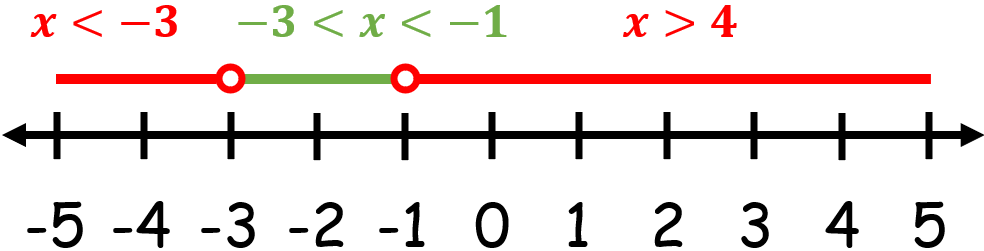Test points: $$\color{red}{x \ = \ -4, \ x \ = \ -2, x \ = \ 0}$$
So, $$\color{red}{⇒ \ x \ < \ -3, \ x \ > \ 4}$$ is the solution.
Solution set: $$\color{red}{\{x \ | \ x \ < \ -3, \ x \ > \ 4\}}$$

6) Solve: $$x^2 \ - \ 1 \ > \ 0$$

$$\color{red}{x_1 \ = \ -1, \ x_2 \ = \ 1}$$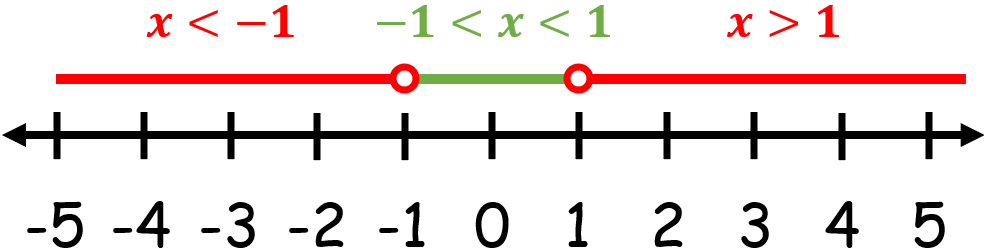Test points:
$$\color{red}{x \ = \ -2, \ x \ = \ 0, x \ = \ 2}$$
So, $$\color{red}{⇒ \ x \ < \ -1, \ x \ > \ 1}$$ is the solution.
Solution set: $$\color{red}{\{x \ | \ x \ < \ -1, \ x \ > \ 1\}}$$

7) Solve: $$x^2 \ - \ 4 \ < \ 0$$

$$\color{red}{x_1 \ = \ -2, \ x_2 \ = \ 2}$$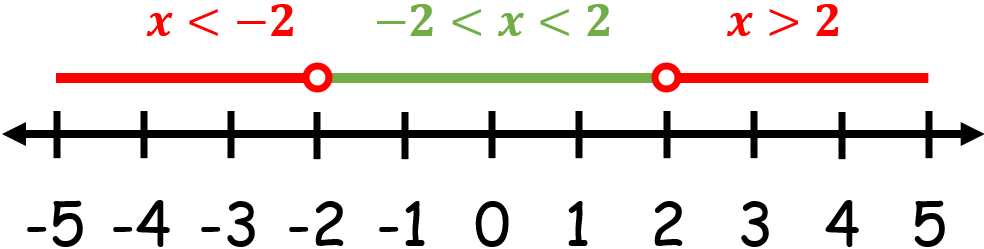Test points:
$$\color{red}{x \ = \ -3, \ x \ = \ 0, x \ = \ 3}$$
So, $$\color{red}{⇒ \ -2 \ < \ x \ < \ 2}$$ is the solution.
Solution set: $$\color{red}{\{x \ | \ -2 \ < \ x \ < \ 2\}}$$

8) Solve: $$(2x \ + \ 2)(3x \ - \ 6) \ < \ 0$$

$$\color{red}{x_1 \ = \ -1, \ x_2 \ = \ 2}$$Test points: $$\color{red}{x \ = \ -2, \ x \ = \ 0, x \ = \ 3}$$
So, $$\color{red}{⇒ \ -1 \ < \ x \ < \ 2}$$ is the solution.
Solution set: $$\color{red}{\{x \ | \ -1 \ < \ x \ < \ 2\}}$$

9) Solve: $$x^2 \ - \ x \ - \ 12 \ > \ 0$$

$$\color{red}{x_1 \ = \ -3, \ x_2 \ = \ 4}$$Test points: $$\color{red}{x \ = \ -4, \ x \ = \ 0, x \ = \ 5}$$
So, $$\color{red}{⇒ \ x \ < \ -3, \ x \ > \ 4}$$ is the solution.
Solution set: $$\color{red}{\{x \ | \ x \ < \ -3, \ x \ > \ 4\}}$$

10) Solve: $$(-x \ - \ 1)(x \ + \ 3) \ > \ 0$$

$$\color{red}{x_1 \ = \ -1, \ x_2 \ = \ -3}$$Test points: $$\color{red}{x \ = \ -4, \ x \ = \ -2, x \ = \ 0}$$
So, $$\color{red}{⇒ \ -3 \ < \ x \ < \ -1}$$ is the solution.
Solution set: $$\color{red}{\{x \ | \ -3 \ < \ x \ < \ -1\}}$$

## Solve Quadratic Inequalities Practice Quiz

### 7th Grade GMAS Math Workbook

$18.99$12.99

### TASC Math Study Guide 2020 – 2021

$20.99$15.99

### FTCE General Knowledge Math for Beginners 2022

$21.99$13.99

### ALEKS Math Full Study Guide

$25.99$12.99